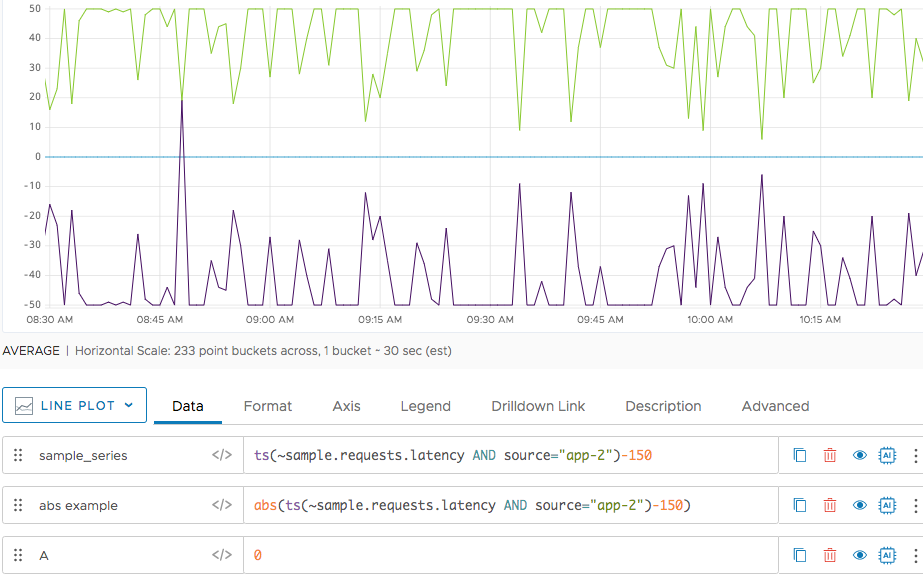Reference to the abs() function

## Summary

``````abs(<tsExpression>)
``````

Returns the absolute value of the time series described by the expression.

## Parameters

ParameterDescription
tsExpression Expression describing the time series for which you want the absolute value.

## Description

The `abs()` function returns the absolute value of each data value in the time series described by the expression. `abs()` returns a separate series of results for each such time series.

If the data points of a time series fluctuate between positive and negative values, you can apply the `abs()` function to display each data point as an absolute value (the distance from zero).

## Examples

Suppose that you have a time series that includes three data points: 2, -1, and -9. If you apply the `abs()` function to the series, these data points are displayed as 2, 1, and 9.

The following example shows how `abs()` displays a corresponding positive value for each negative value in the chart. We’ve included a line for 0 to illustrate how the function works.